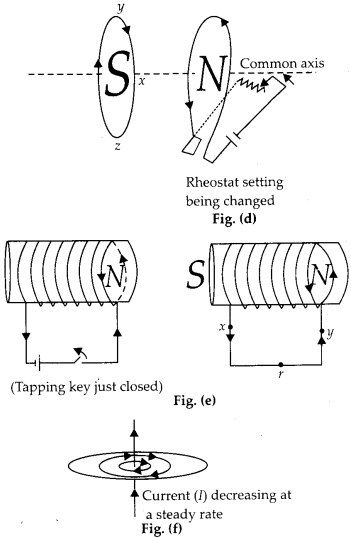Enlightened

# Question 1: NCERT Solutions for 12th Class Physics: Chapter 6-Electromagnetic Induction

• 0

Question 1: NCERT Solutions for 12th Class Physics: Chapter 6-Electromagnetic Induction

Predict the direction of induced current in the situations described by the following Figures (a) to (f).Share

1. Solution:
Direction of induced current in all the situations shown above can be decided in the light of Lenz’s law.Fig. (a) : South pole is moving closer, so the current is clockwise in the end of solenoid closest to magnet.
Fig. (b) : Following Lenz’s law, the current flow anticlockwise in the loop at the left and clockwise in the loop at the right.
Fig. (c) : Inner side of loop-1 become south pole whose strength increasing with increase in current. So the inner side of loop should also become south pole according to Lenz’s law.
Fig. (d) : Current is decreasing with increase in rheostat, so North pole is getting weaker, the current in inner part of loop-1 will flow clockwise.
Fig. (e) : Induced current in the right coil is from X to Y,
Fig. (f) : No induced current since magnetic lines of force are in the plane of the loop.

Check the complete chapter with solutions.

NCERT Solutions for 12th Class Physics: Chapter 6-Electromagnetic Induction

• 0